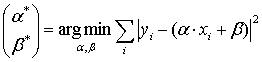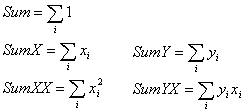On Time, On Point, On Budget!

# Pattern Accumulator

### Problem description

Articles about different patterns usually begin with pattern’s general scheme. Further, the author of article shows the particular usage of a pattern, as an example. Let me break this tradition and begin with a specific problem, which can be found in business applications very often.

Let’s assume that your business application has grown up to that point, where the client requires functionality of some reports output. I do not mean the records, where the main part is a tabular part. Now I consider consolidated statistical report where each indicator is calculated by using the whole data sample.

Like all normal people, I did something like this:

1. Design the structure (class) with the fields (properties), which values will be displayed in the report.
2. Do data sample, which is used in creation of report (for example, the inquiry to the database).
3. Pass each data line (element, object) with foreach and summarize results in the appropriate fields of our structure.
4. Generate presentation on the screen directly regarding to completed copy of the structure (class).

Of course, inquiry to the database can be written so, that all the summation will be performed on the database server. But now, is not that important, let’s assume that the data lines passage should be done on the client side.

Below is a sample of code, implementing such a scenario.

/// <summary>
/// </summary>

public interface Client
{
/// <summary>
/// Group number (clients are grouped)
/// </summary>
int Group {get;}

/// <summary>
/// Some real option.
/// For example, customer’s account.
/// </summary>
double AnyDoubleValue {get;}
}

/// <summary>
/// Structure of summary indicators.
/// </summary>
public struct SummaryReport
{
public int TotalCount;
public int TotalCountInGroup1;
public int TotalCountInGroup2;
public int TotalCountInGroup3;

public double MeanAnyDoubleValue;
public double MinAnyDoubleValue;
public double MaxAnyDoubleValue;
//...
/// <summary>
/// Update summary indicators for client’s data sample.
/// </summary>
/// <param name="clients"></param>
public void Update(ICollection<Client> clients)
{
TotalCount = 0;
TotalCountInGroup1 = 0;
TotalCountInGroup2 = 0;
TotalCountInGroup3 = 0;
MeanAnyDoubleValue = 0;
MinAnyDoubleValue = double.MaxValue;
MaxAnyDoubleValue = double.MinValue;
foreach(Client client in clients)
{
TotalCount++;
switch(client.Group)
{
case 1:
TotalCountInGroup1++;
break;
case 2:
TotalCountInGroup2++;
break;
case 3:
TotalCountInGroup3++;
break;
}
if (client.AnyDoubleValue < MinAnyDoubleValue)
MinAnyDoubleValue = client.AnyDoubleValue;
if (client.AnyDoubleValue > MaxAnyDoubleValue)
MaxAnyDoubleValue = client.AnyDoubleValue;
MeanAnyDoubleValue += client.AnyDoubleValue;
}
if (TotalCount > 0)
MeanAnyDoubleValue /= TotalCount;
}
}

I wonder, how many readers would say: “Yes, damn it, I have written it many times, and I’m awfully tired of this!” when they see the SummaryReport.Update method?
For those, who do not think so, I’ll try to bring all the suffering of human, who have said these words. See, the code is really clean and simple. But, what if you need to implement more than one such report, for example 4-5 records with almost the same summation algorithm. How many times are you ready to write “if” operator to search the maximum (minimum) value until you’ll be finally fed up? Isn’t it irritating to write almost the same SummaryReport’s structures? I think it’s time to finish it, and pattern Accumulator will help us with it.

### Problem Solving – Accumulator

Well, we’ve all realized that we no longer want to write the search of the minimum values in the cycle. More precisely, we want to write this code just once, in convenient form so as to use it later. The idea is the following – instead of writing every time structures like SummaryReport, we create classes that can summarize data for a particular, specific algorithm for each such class. We’ll call such classes accumulators (of data) and in the name of these classes will add postfix Accumulator. It will be better even to design at once the IAccumulator interface, which will implement classes-accumulators.

/// <summary>
/// IAccumulator
/// </summary>
public interface IAccumulator<ValueType>
{
/// <summary>
/// Reset accumulation
/// </summary>
void Reset();

/// <summary>
/// </summary>
/// <param name="value"></param>
}

I think that you’ve already understood the purpose of methods: Reset – resets the stored total data, Add – handles the new data (for example, adds to the sum new value). Isn’t it very similar to interface IEnumerator ?
Now let’s try to implement value accumulator, which will carry on the counter of elements (i.e., demand Add method), the total sum, and at the same time it will refresh the minimum maximum value.

/// <summary>
/// Value accumulator
/// </summary>
/// <typeparam name="ValueType"></typeparam>
public class ValueAccumulator<ValueType> : IAccumulator<ValueType>
where ValueType : new()
{
long totalCount;
ValueType totalSumm;
bool firstValue;
ValueType minValue;
ValueType maxValue;

public ValueAccumulator()
{
Reset();
}

public ValueType TotalSumm
{
}

public ValueType MeanValue
{
get
{
return (totalCount > 0) ?
totalSumm / totalCount :
totalSumm;
}
}

public ValueType MaxValue
{
get { return maxValue; }
}

public ValueType MinValue
{
get { return minValue; }
}

public void Reset()
{
firstValue = true;
totalCount = 0;
totalSumm = new ValueType();
minValue = new ValueType();
maxValue = new ValueType();
}

{
totalCount++;
totalSumm += value;
if (firstValue)
{
firstValue = false;
minValue = value;
maxValue = value;
}
else
{
if (minValue > value)
{
minValue = value;
}
if (maxValue < value)
{
maxValue = value;
}
}
}
}

I did everything to make the code above (generic ValueAccumulator) being compiled. But unfortunately, it is impossible to do this: generics can’t be explained that operators +=,/,<,> are implemented in ValueType.

That fact that generics don’t support a more detailed description of their own type-parameters, was the sad news for me. In C++ patterns, for example, such problems would not ever arise. However, there is no strong typing in C++. I am sure, that C# generics developers are aware of this problem and, certainly, they have an answer.

There are several ways out:
1. Refuse ValueAccumulator class-generic and realize, for example, DecimalValueAccumulator, which implements IAccumulator interface.
2. Pass an interface in the constructor of ValueAccumulator class, which incurs functions of =, <,> operators.

In order not to go too far from the topic of article – let’s choose the first way. You will find Class Code DecimalValueAccumulator in the application; it is obtained by replacing the ValueType type-setting to type decimal in the code mentioned above.

So, the time has come, when one variable structure of SummaryReport can be replaced by some copies of DecimalValueAccumulator.

public static void Update(
ICollection<Client> clients,
DecimalValueAccumulator accForGroup1,
DecimalValueAccumulator accForGroup2,
DecimalValueAccumulator accForGroup3,
DecimalValueAccumulator accTotal
)
{
foreach (Client client in clients)
{
switch (client.Group)
{
case 1:
break;
case 2:
break;
case 3:
break;
}
}
}

Here are those good things that we have after all we’ve done:
1.we no longer see the “primitive” code such as counting the total sum, the total number, search of minimum, maximum in the Update method;
2. now, with less code, we have a much more detailed statistical information, than it was in old SummaryReport’s structure (now each group has it’s total number, sum, minimum, maximum, an average of property setting Client.AnyDoubleValue);
3. DecimalValueAccumulator – semantically is not tied to a subject side of application, and therefore can be reused in other project.

But, there is something that I do not like:

1. still, there is a foreach, which is not desirable to write at every time when we use accumulators;
2. switch operator, which redirects values in different accumulators, is not desirable to write too, if it is possible.

But, this what the next paragraph is about.

### Combined accumulators

Idea of combining accumulators means the following – create classes of accumulators, which redirect the accumulated value to other accumulators. It would be nice, if such accumulator would be able to perform intermediate conversion of accumulated value.

Below is a public interface of class CollectionAccumulator. CollectionAccumulator – collection accumulator, which can save us from writing the foreach operator at every time when we need to go through the collection and apply the second accumulator to the elements.

/// <summary>
/// CollectionAccumulator
/// </summary>
public class CollectionAccumulator : IAccumulator<IEnumerable>
{

public CollectionAccumulator(
IAccumulator valueAcc,
Converter<object, object> valueConvertor
);

public IAccumulator ValueAccumulator
{
get;
}

public Converter<object, object> ValueConvertor
{
get;
}

public void Reset();

public static void Accumulate(IAccumulator valueAcc, IEnumerable collection);

public static void Accumulate<ValueType>( IAccumulator<ValueType> valueAcc, ICollection<ValueType> collection);

public static void Accumulate(
IAccumulator valueAcc,
IEnumerable collection,
Converter<object, object> valueConvertor
);

public static void Accumulate<ValueType>(
IAccumulator<ValueType> valueAcc,
IEnumerable collection,
Converter<object, ValueType> valueConvertor
);
}

Now I will tell you about the next accumulator. It will help us to get rid of switch operators in the code.

/// <summary>
/// SeparateAccumulator
/// </summary>
public class SeparateAccumulator<ObjectType, ValueType> :  IAccumulator<ObjectType>, IAccumulator
{
public SeparateAccumulator(
Converter<ObjectType, object> separateMethod,
Converter<ObjectType, ValueType> valueConvertor,
params KeyValuePair<object, IAccumulator<ValueType>>[] keyedAccumulators);

public Dictionary<object, IAccumulator<ValueType>> AccumulatorsByKey
{
get;
}

public void Reset();

}

The value conversion to the correct accumulator is made by delegate separateMethod, which returns the key, on which we remove accumulator from hash-table.

The code of our Update method, with usage of classes-accumulators, mentioned above, will look like this:

public static void Update(
ICollection<Client> clients,
DecimalValueAccumulator accForGroup1,
DecimalValueAccumulator accForGroup2,
DecimalValueAccumulator accForGroup3,
DecimalValueAccumulator accTotal
)
{
Converter<object, decimal> getAnyDoubleValue = delegate(object client)
{
return (decimal)(client as Client).AnyDoubleValue;
};
Converter<object, object> getGroup =
delegate(object client)
{
return (client as Client).Group;
};

SeparateAccumulator<object, decimal> separateAcc =
new SeparateAccumulator<object, decimal>(
getGroup,
getAnyDoubleValue,
new KeyValuePair<object, IAccumulator<decimal>>(1, accForGroup1),
new KeyValuePair<object, IAccumulator<decimal>>(2, accForGroup2),
new KeyValuePair<object, IAccumulator<decimal>>(3, accForGroup3)
);

CollectionAccumulator.Accumulate(separateAcc, clients);
CollectionAccumulator.Accumulate<decimal>(accTotal,
clients, getAnyDoubleValue);
}

I can’t say that with the use of combined accumulators Update method has become easier or shorter, it doesn’t, and in the specific example I would prefer to write once again the foreach operator, than to apply class CollectionAccumulator.

But it is doesn’t mean that we have wasted our time. First, our example of accumulators’ application is too simple to see its benefit from combined accumulators. Secondly, the combined accumulators are more abstract essences, and therefore they are more difficult for perception. A difficulty in perception can justify itself only in more complex tasks. And thirdly, it is also a matter of habit to apply one or another tool.

### Accumulators in mathematical calculations

A good pattern is useful at coding programs of different subject areas. I have started the article from example of accumulators usage in “accounting” program. Accumulators, as I think, more useful for coding mathematical calculations and solving algorithmic problems.

#### Method of mean squares

I will start with a simple example – method of mean squares. This method is known among probably everyone, who have studied physics and mathematics or have “computer-related” education.Figure 1 shows the mathematical formulation of the most simple case of mean square method. I will not give here a formula for getting the optimal values of alpha and beta, the reader will certainly be able to do it. Let me just say that to calculate the optimal solution it is necessary to calculate the following sums first:If the sums (2) divide by number (i.e., get averaged), we’ll get such thing, that in mathematical statistics called moments (correct me, if I am not right). But, no matter how they’re called, it is necessary to calculate them. And, as the reader may have guessed, the most right place to do it – accumulator.

The method, in which the method of mean squares will be realized, will accept accumulators with sums and will fulfill remained calculations. Below I result only a code of classes’ public interfaces, a complete code the reader will find in application.

Do you like the article and want us to develop your project? Feel free to contact us here!

This entry was posted on Sunday, January 17th, 2010 at 5:16 am and is filed under Architecture.